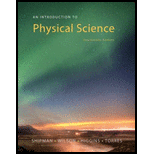Chapter 1, Problem 19SAAn Introduction to Physical Science

14th Edition
James Shipman + 3 others
ISBN: 9781305079137

Solutions

Chapter
SectionAn Introduction to Physical Science

14th Edition
James Shipman + 3 others
ISBN: 9781305079137
Textbook Problem

What are the four most common metric prefixes?

To determine
Four common metric preifixes.

Explanation

A series of metric prefixes is used to express the multiples of 10.

Four most common metric prefixes are:

mega=1,000,000(million, 106)kilo=(thousand, 103)

Still sussing out bartleby?

Check out a sample textbook solution.

See a sample solution

The Solution to Your Study Problems

Bartleby provides explanations to thousands of textbook problems written by our experts, many with advanced degrees!

Get Started